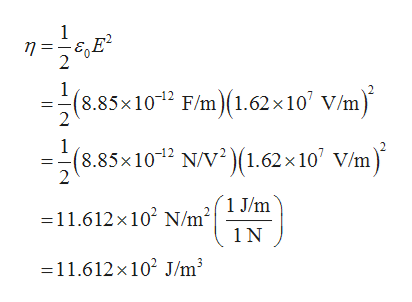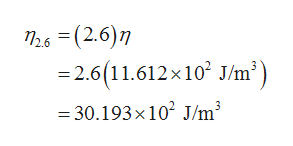Part a and b Please.Polystyrene has dielectric constant 2.6 and dielectric strength 2.0×10^7 V/m. A piece of polystyrene is used as a dielectric in a parallel-plate capacitor, filling the volume between the plates.Part A When the electric field between the plates is 81 % of the dielectric strength, what is the energy density of the stored energy?Part B When the capacitor is connected to a battery with voltage 500.0 V, the electric field between the plates is 81 % of the dielectric strength. What is the area of each plate if the capacitor stores 0.20 mJ of energy under these conditions?

Question

Part a and b Please.

Polystyrene has dielectric constant 2.6 and dielectric strength 2.0×10^7 V/m. A piece of polystyrene is used as a dielectric in a parallel-plate capacitor, filling the volume between the plates.

Part A
When the electric field between the plates is 81 % of the dielectric strength, what is the energy density of the stored energy?

Part B
When the capacitor is connected to a battery with voltage 500.0
V, the electric field between the plates is 81 % of the dielectric strength. What is the area of each plate if the capacitor stores 0.20 mJ of energy under these conditions?

Step 1

(Part A)

The electric field strength between the plates with 81% of dielectric strength can be calculated as,

Step 2

Write the expression for the energy density of the stored energy.help_outlineImage Transcriptionclose1 2 (8.85x102 F/m(1.62x10 V/m) 1 (8.85x1012 N/V2)(1.62x 10 V/m) =11.612 x102 N/m°{ 1 J/m 1 N 11.612x 102 J/m3 fullscreen
Step 3

The energy density of the stored energy in polystyrene capacit...help_outlineImage TranscriptioncloseT26 (2.6) 2.6 (11.612x10 J/m3 30.193x 102 J/m3 fullscreen

Want to see the full answer?

See Solution

Want to see this answer and more?

Our solutions are written by experts, many with advanced degrees, and available 24/7

See Solution
Tagged in

Electric Charges and Fields